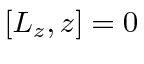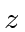## Sample Test Problems

1. Assume an electron is bound to a heavy positive particle with a harmonic potential. Calculate the energy shifts to all the energy eigenstates in an electric field(in thedirection).

2. Find the energies of thehydrogen states in a strong uniform electric field in the z-direction. (Note, since spin plays no role here there are just 4 degenerate states. Ignore the fine structure corrections to the energy since the E-field is strong. Remember to use the fact that. If you are pressed for time, don't bother to evaluate the radial integrals.)

3. An electron is in a three dimensional harmonic oscillator potential. A small electric field, of strength, is applied in thedirection. Calculate the lowest order nonzero correction to the ground state energy.

4. Hydrogen atoms in thestate are put in a strong Electric field. Assume that the 2s and 2p states of Hydrogen are degenerate and spin is not important. Under these assumptions, there are 4 states: the 2s and three 2p states. Calculate the shifts in energy due to the E-field and give the states that have those energies. Please work out the problem in principle before attempting any integrals.

Jim Branson 2013-04-22